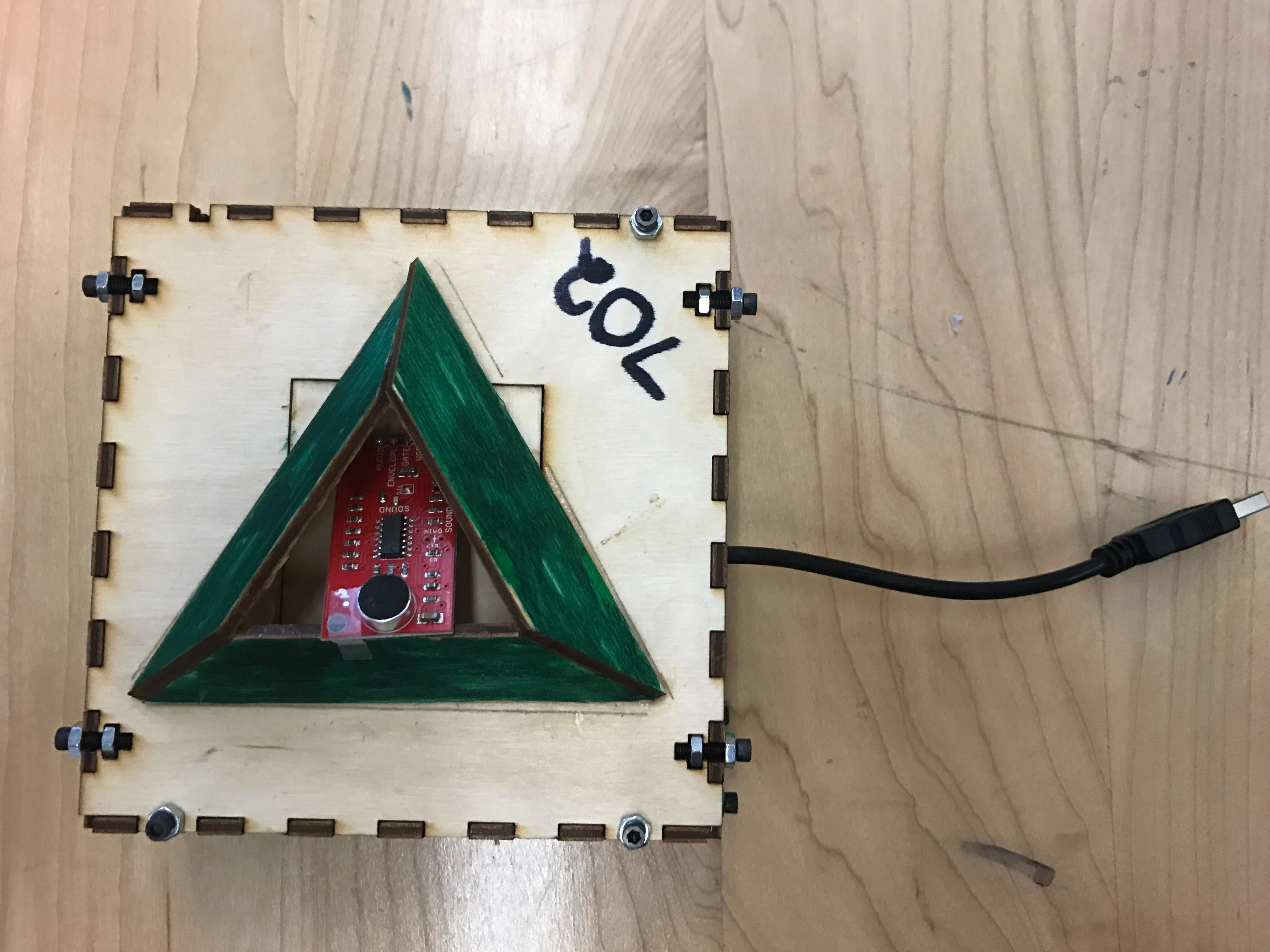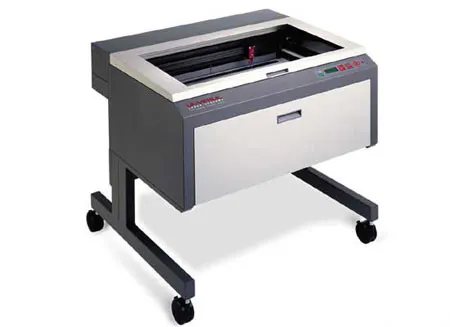# Group 702 Sound Sensor Project

Our project detects sound and the data is collected so that we can measure at what points in the day has the highest amount of volume.

IntermediateFull instructions provided20 hours464## Things used in this project

### Hardware componentsParticle Photon
×1×1Jumper wires (generic)
×1

### Hand tools and fabrication machinesLaser cutter (generic)

## Code

### Sound Code for Photon

Arduino
```const long sample_rate = 50; // time between samples (in miliseconds)
const int array_size = 1200; // 1000/50=20 * 60=1200
int snd_array[array_size] = {};
int snd_max, prev_max = 0;
int snd_min, prev_min = 4096;
double snd_avg = 2048;

// Shift all the values right by 1
for(int i = array_size-1; i >= 1; i--)
{
snd_array[i] = snd_array[i-1];
if((snd_array[i] < snd_min) && (snd_array[i] != 0))
{
snd_min = snd_array[i];

}
if(snd_array[i] > snd_max)
{
snd_max = snd_array[i];

}
}

snd_array = value;

// Average array
float avg_sum = 0;
int size = 0 ;
for (int a=0; a <= array_size; a++)
{
if(snd_array[a] > 0)
{
size++ ;
avg_sum  = avg_sum + snd_array[a];
}
}
snd_avg = avg_sum / size;
}

digitalWrite(D7, HIGH);
} else {
digitalWrite(D7, LOW);
}
}

unsigned long now = millis();
Serial.print("Avg: "); Serial.println(snd_avg);
Serial.print("Min: "); Serial.println(snd_min);
Serial.print("Max: "); Serial.println(snd_max);
snd_avg = 0;
snd_min = 4096;
snd_max = 0;
//snd_array[array_size] = {};
}
}

void setup() {
Serial.begin(9600);
pinMode(A0, INPUT); // mic AUD connected to Analog pin 0
pinMode(D7, OUTPUT); // flash on-board LED

Particle.variable("snd_avg", snd_avg);
Particle.variable("snd_max", snd_max);
Particle.variable("snd_min", snd_min);

}

void loop() {

delay(sample_rate);
}
```

## Credits

### Blake Trendel

1 project • 2 followers

### Michael Herrera

1 project • 1 follower

### sanee kassam

1 project • 2 followers
YA YA YA